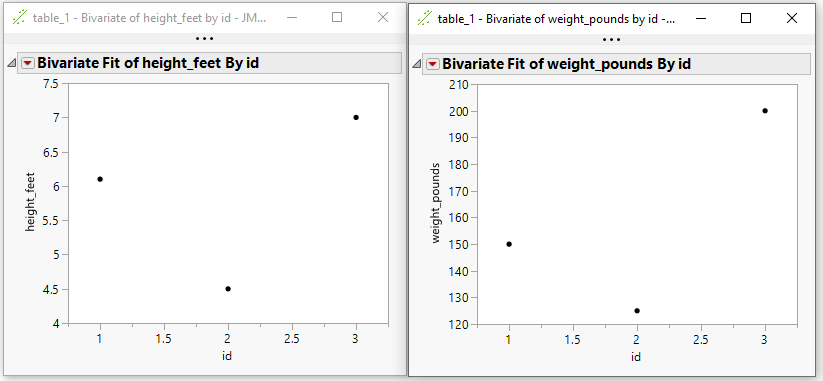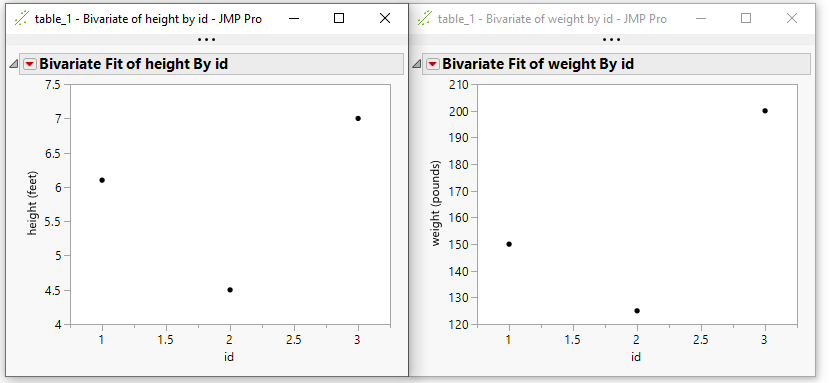Our World Statistics Day conversations have been a great reminder of how much statistics can inform our lives. Do you have an example of how statistics has made a difference in your life? Share your story with the Community!
Choose Language Hide Translation Bar
Highlighted

## Update Column Name from Configuration File Using JSL

Hi JMP users,

I would like to update column names from a configuration file using JSL and make plots which will have the updated column names in their titles and axes. I am looking for ideas on how I can do this.

I am attaching my code so far and tables. Thanks for the help.

I have attached what I would like the final graph to look like (desired_Bivariate)

-Simon

``````Names Default To Here( 1 );
dt = Open( "table_1.jmp" );
dt1 = Open( "config_table.jmp" );
dt1 << New Column( "New Column", character, formula( Column_name || "_" || additional_name ) );

graph = dt << Bivariate( Y( :height ), X( :id ) );``````

2 ACCEPTED SOLUTIONS

Accepted Solutions
Highlighted

## Re: Update Column Name from Configuration File Using JSL

Try this:

``````Names Default To Here( 1 );
dt = Open( "table_1.jmp" );
dt1 = Open( "config_table.jmp" );

for(i = 1, i <= N Rows(dt1), i++,
x = Column(dt1, "Column_name")[i];
Column(dt, i) << Set Name( x || "_" || y);
);``````
Highlighted

## Re: Update Column Name from Configuration File Using JSL

Here are 2 different approaches to the issue that do not change the actual name of the columns, but accomplishes what you are asking for.  The first one creates Transform columns that have the new names specified for the run of the bivariate.  Those columns only exist for the bivariate execution.The second example uses the Units Column Property for the different columns in the data table.  The platforms in JMP honor the Units column property, and display it as an addition to the actual column name by placing the Units value in () following the column name.....(i.e. Height(feet) )``````// This version of the script used Transform variables
// to temporarially change the name of the column just
// for the running of the Bivariate

Names Default To Here( 1 );
dt = Data Table( "table_1" );
dt1 = Data Table( "config_table" );

// Loop across the columns to run the Bivariate
For( i = 2, i <= N Cols( dt ), i++,
// Check to see if a column name change is specified
// in the config table
foundRow = dt1 << get rows where(
:Column_name == Column( dt, i ) << get name & dt1
);
// If a column name change is found make the change
If( N Rows( foundRow ) > 0,
Eval(
Substitute(
Expr(
Bivariate(
Y(
Transform Column(
__trans__,
Formula( __col__ )
)
),
X( :id )
)
),
Expr( __trans__ ),
dt1:Column_name[foundRow] || "_" || dt1
Expr( __col__ ),
Parse( ":" || dt1:Column_name[foundRow] )
)
),
// otherwise just run the bivariate
dt << Bivariate( Y( Column( i ) ), X( :id ) )
);
);``````
``````// This version of the script takes advantage of the Units
// column property.  It will automatically add (unit value) to
// the display output, without actually changing the columns
// name
Names Default To Here( 1 );
dt = Data Table( "table_1" );
dt1 = Data Table( "config_table" );

// Add the Units Column Property to the columns in the
// original data table
For( i = 1, i <= N Cols( dt ), i++,
foundRow = dt1 << get rows where(
:Column_name == Column( dt, i ) << get name & dt1
);

If( N Rows( foundRow ) > 0,
column( dt, i ) << set property("units", eval(dt1:additional_name[foundRow]));
);
);

// Now run the normal bivariates
For( i = 2, i <= N Cols( dt ), i++,
dt << Bivariate( Y( Column( i ) ), X( :id ) )
);

``````

Jim
4 REPLIES 4
Highlighted

## Re: Update Column Name from Configuration File Using JSL

Try this:

``````Names Default To Here( 1 );
dt = Open( "table_1.jmp" );
dt1 = Open( "config_table.jmp" );

for(i = 1, i <= N Rows(dt1), i++,
x = Column(dt1, "Column_name")[i];
Column(dt, i) << Set Name( x || "_" || y);
);``````
Highlighted

## Re: Update Column Name from Configuration File Using JSL

Great. Thanks ThuongLe.

Highlighted

## Re: Update Column Name from Configuration File Using JSL

Here are 2 different approaches to the issue that do not change the actual name of the columns, but accomplishes what you are asking for.  The first one creates Transform columns that have the new names specified for the run of the bivariate.  Those columns only exist for the bivariate execution.The second example uses the Units Column Property for the different columns in the data table.  The platforms in JMP honor the Units column property, and display it as an addition to the actual column name by placing the Units value in () following the column name.....(i.e. Height(feet) )``````// This version of the script used Transform variables
// to temporarially change the name of the column just
// for the running of the Bivariate

Names Default To Here( 1 );
dt = Data Table( "table_1" );
dt1 = Data Table( "config_table" );

// Loop across the columns to run the Bivariate
For( i = 2, i <= N Cols( dt ), i++,
// Check to see if a column name change is specified
// in the config table
foundRow = dt1 << get rows where(
:Column_name == Column( dt, i ) << get name & dt1
);
// If a column name change is found make the change
If( N Rows( foundRow ) > 0,
Eval(
Substitute(
Expr(
Bivariate(
Y(
Transform Column(
__trans__,
Formula( __col__ )
)
),
X( :id )
)
),
Expr( __trans__ ),
dt1:Column_name[foundRow] || "_" || dt1
Expr( __col__ ),
Parse( ":" || dt1:Column_name[foundRow] )
)
),
// otherwise just run the bivariate
dt << Bivariate( Y( Column( i ) ), X( :id ) )
);
);``````
``````// This version of the script takes advantage of the Units
// column property.  It will automatically add (unit value) to
// the display output, without actually changing the columns
// name
Names Default To Here( 1 );
dt = Data Table( "table_1" );
dt1 = Data Table( "config_table" );

// Add the Units Column Property to the columns in the
// original data table
For( i = 1, i <= N Cols( dt ), i++,
foundRow = dt1 << get rows where(
:Column_name == Column( dt, i ) << get name & dt1
);

If( N Rows( foundRow ) > 0,
column( dt, i ) << set property("units", eval(dt1:additional_name[foundRow]));
);
);

// Now run the normal bivariates
For( i = 2, i <= N Cols( dt ), i++,
dt << Bivariate( Y( Column( i ) ), X( :id ) )
);

``````

Jim
Highlighted

## Re: Update Column Name from Configuration File Using JSL

Awesome. Thanks Jim.

Article Labels